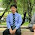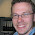05 August 2015

Rounding Amounts, the missing cent

Dividing a certain amount over several rows can be quite tricky, simply rounding can lead to differences.
Let me try to explain what I mean. When you need to divide 100 by 3, the answer is 33.333333333333 (and a lot more threes).
Money only goes to cents, so if each one gets 33.33, there is a cent missing. (3 times 33.33 equals 99.99)
To solve this cent-problem, we decide that the difference should be added (or subtracted) on the last row.

To create an example, first let's create a table with only three records in it.

```   SQL> create table t
2  as
3  select rownum + 42 id
4    from dual
5  connect by level <= 3
6  ;

Table created.
SQL> select *
2    from t
3  /

ID
----------
43
44
45
```

In the code below the amount that we want to divide is included in the query on line 2. On line 3 the analytic counterpart of the COUNT(*) function is used to determine the number of records in the resultset. On line 4 you can see the result when you round the amount divided by the number of records in the resultset. All records show 33.33, just as we expected.
Line 5 shows a trick using the LEAD function to identify the last record.

```      SQL> select id
2      ,100 amount
3      ,count(*) over () entries
4      ,round (100 / count(*) over (), 2) rounded
5      ,lead (null, 1, 'x') over (order by id) lastrow
6    from t
7  /

ID            AMOUNT    ENTRIES    ROUNDED L
---------- ---------- ---------- ---------- -
43       100           3      33.33
44       100           3      33.33
45       100           3      33.33 x
```

Because we identified the last record in the resultset, it is easy to calculate the difference between the amount that we want to divide and the total of the rounded amount.
In the code below this is done on lines 6 through 9. In plain English it reads: "Take the rounded amount and add to that the difference between the amount and the sum of the rounded amount, but only if you're on the last record"

```SQL> select id
2      ,amount
3      ,entries
4      ,rounded
5      ,sum (rounded) over (order by id) running_rounded
6      ,rounded + case
7          when lastrow = 'x'
8          then amount - sum (rounded) over (order by id)
9          else 0 end final_amount
10    from (
11  select id
12      ,100 amount
13      ,count(*) over () entries
14      ,round (100 / count(*) over (), 2) rounded
15      ,lead (null, 1, 'x') over (order by id) lastrow
16    from t
17  )
18  /

ID     AMOUNT    ENTRIES    ROUNDED RUNNING_ROUNDED FINAL_AMOUNT
---------- ---------- ---------- ---------- --------------- ------------
43        100          3      33.33           33.33        33.33
44        100          3      33.33           66.66        33.33
45        100          3      33.33           99.99        33.34
```
As you can see in the result, the missing cent is added to the last record.
Looking at the query again, I realize that it is not necessary to use the ORDER BY in the SUM function.

1.What if for example i have 42 records and i wish to divide 100 by 42. I would get a rounded value of 2.38. If i multiply this by 42 it would amount to just 99.96. What if want to spread the .04 difference on 4 records, not just the last record. In effect i'll be having 4 records with 2.39. Right now i'm doing this via cursor. Im kinda hoping i can do this using sql or analytic functions

1.You could do something like:

with t as
(select rownum id
from dual
connect by level <= 42
)
select id
,rounded +
case when rownum <= (amount - (entries * rounded)) * 100
then 0.01
else 0
end final_amount
from (
select id
,100 amount
,count(*) over () entries
,round (100 / count(*) over (), 2) rounded
from t
)

2.There is a better solution here: http://nuijten.blogspot.nl/2016/05/rounding-amounts-divide-cents-over.html
I liked your question, so created a new blogpost out of it :)